# Scripting Languages and NI LabVIEW

## Scripting Languages and LabVIEW

In the scientific and academic community, scripting languages are commonly use for analysis and visualization. NI LabVIEW software offers different options for using scripting languages, such as The MathWorks, Inc. MATLAB® software (.m file scripts), CGI, XML, Xmath, Scilab from the Scilab Consortium (Digiteo), and others within the LabVIEW development environment in combination with G graphical programming. Many of the most popular scripting languages (Python, PHP, Perl, Tcl, and so on) on the market offer different methods of connectivity such as a C language API, and there is always a way to integrate them with LabVIEW.

### What is a Scripting Language?

A common definition is that “a scripting language, script language, or extension language is a programming language that controls a software application” . Programs written with a scripting language are usually command-based and interpreted, not compiled, a reason for their typically being slower than programs created in C, C++, G, and other programming languages that execute compiled and optimized code. Scripting languages usually follow a specific syntax, and rules are not very flexible in some cases. However, because these scripting languages are text-based and many of them are based on open standards that use simple rules and commands for executing complex tasks, they have a space in the academic and scientific community. They are also very popular in Web page creation and administration, so computer science and IT professionals commonly use them in Web-related projects.

Consider the options available within the LabVIEW development environment, which allow a developer to use a hybrid approach when creating code: the combination of the G graphical programming language with text-based commands or scripts. These are usually implemented within the LabVIEW development environment through a node in which inputs and outputs, or variables or constants, are defined to connect the node with the G programming language. Within the node, you can use a set of script commands to acquire, analyze, or visualize data. The basic architecture of a script node in LabVIEW is shown in Figure 1. Usually, inputs and outputs are optional but recommended to have some level of interactivity with LabVIEW and the G programming language.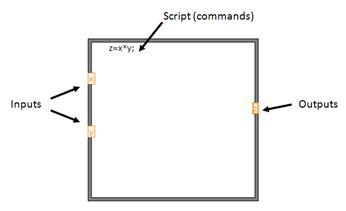Figure 1. Basic “Architecture” of a Script Node in LabVIEW

### Node Options for Using Scripting Language in LabVIEW

You can choose from the following six options to use scripting languages within the LabVIEW development environment:

• Formula Node
• Expression Node
• LabVIEW MathScript Node
• Xmath script node (requires NI MATRIXx)
• MATLAB script node (requires MATLAB)
• Scilab script node (requires Scilab and the LabVIEW-Scilab Gateway Software)

You probably use script nodes to execute math scripts in LabVIEW. The LabVIEW MathScript, MATLAB, Scilab, and Xmath script nodes are similar to the Formula Node, but you can use them to import an existing LabVIEW MathScript script written in the MATLAB language syntax or an existing Xmath script in ASCII form and run the script in LabVIEW. As with a Formula Node, you can pass data to and from the node.

#### Expression Node

Use an Expression Node to calculate expressions that contain a single variable. Expression Nodes are useful when an expression has only one variable but is otherwise complicated.

Expression Nodes use the value you pass to the input terminal as the value of the variable. The output terminal returns the value of the calculation. Expression Nodes might not be considered a scripting language, but you can use a small subset of functions and operators directly on the wires that connect the nodes in G. The Expression Node is included here because it is a text-based expression option available in LabVIEW.

Consider this simple expression:

x * x + 33 * (x + 5)

The following block diagram uses numeric functions to represent this expression.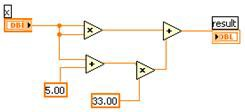Figure 2. Using Numeric Functions to Represent an Expression

You can simplify this code drastically using an Expression Node. The following code provides the same functionality as the previous block diagram: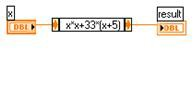Figure 3. Using Expression Node to Simplify Code in Figure 2

Expression Nodes support some polymorphism. The input terminal of an Expression Node is the same data type as the control or constant you wire to it. The output terminal is the same data type as the input terminal. The data type of the input can be any noncomplex scalar number, array of noncomplex scalar numbers, or cluster of noncomplex scalar numbers. With arrays and clusters, the Expression Node applies the expression to each element of an input array or cluster.

#### Formula Node

Formula Nodes are much more extensive and powerful than Expression Nodes and also might not be considered a scripting language because they are more oriented toward formula solving and limited to a set of functions and operators. However, Formula Nodes are included in this list because of their similarities to other scripting languages (and with the C language syntax) and because it is a convenient text-based node that you can use to perform mathematical operations on the LabVIEW block diagram.

You do not have to access any external code or applications, and you do not have to wire low-level arithmetic functions to create equations. In addition to text-based equation expressions, the Formula Node accepts text-based versions of if statements, while loops, for loops, and do loops, which are familiar to C programmers. These programming elements are similar to those you find in C programming but are not identical. Formula Nodes are useful for equations that have many variables or are otherwise complicated and for using existing text-based code. You can copy and paste the existing text-based code into a Formula Node rather than recreating it graphically.

Formula Nodes use type checking to make sure that array indexes are numeric data and that operands to the bit operations are integer data. They also check to make sure array indexes are in range. For arrays, an out-of-range value defaults to zero and an out-of-range assignment defaults to indicate no operation occurs. Formula Nodes also perform automatic type conversion. The Formula Node, shown in Figure 4, is a resizable box similar to the For Loop, While Loop, Case Structure, Stacked Sequence structure, and Flat Sequence structure. However, instead of containing a subdiagram, the Formula Node contains one or more C-like statements delimited by semicolons, as in the following example. As with C, you can add comments by enclosing them inside a slash/asterisk pair (/*comment*/) or by preceding them with two slashes (//comment).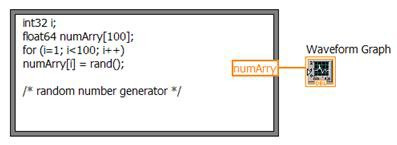Figure 4. Formula Node Example

In Figure 4, the code defines an integer (i) and an array (numArry); then, a for loop used with the rand function generates 100 random numbers. Finally, LabVIEW plots the output values in a waveform graph, as shown in the following front panel.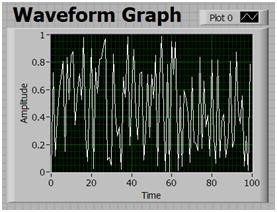Figure 5. Data Generated by the Formula Node Script

There is no limit to the number of variables or equations allowed in a Formula Node, and you can declare and use a variable inside the Formula Node without relating it to an input or output wire. Variables can be floating-point numeric scalars whose precision depends on the configuration of your computer. You also can use integers and arrays of numeric values for variables. One point to consider is that the Formula Node does not support complex data types or the matrix data type. Refer to the Formula Node and Expression Node Functions topic in the LabVIEW Help (linked below) for extensive list of commands and functions supported in the Formula Node.

The following code allows you to convert readings from a thermocouple type J (Iron-Constantan), one of the most common thermocouples along with type K thermocouple, and convert it to either the Celsius (C) or Fahrenheit (F) scale.

Table 1 summarizes the voltage-to-degrees coefficients for the NIST polynomial equation:

T = a0 + a1V + a2V2 + ... + anVn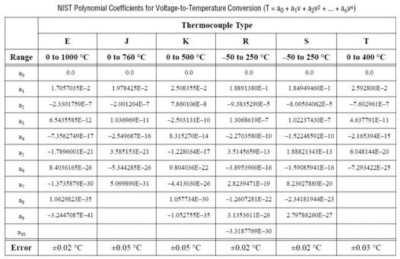Table 1. Voltage-to-Degrees Coefficients for the NIST Polynomial Equation

For simplicity and demonstration purposes, the following Formula Node implementation focuses only on the 0 to 76 °C temperature range and the conversion of the voltage (mV) generated by the thermocouple, ignoring the cold-junction compensation (CJC) reading from a different source (usually a thermistor or IC).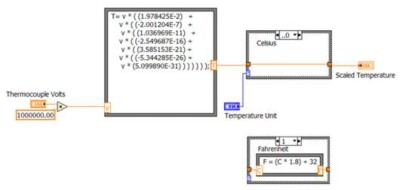Figure 6. Formula Nodes Used for Linearization of Type J Thermocouple Readings

As shown in Figure 6, two Formulas Nodes are used, one for the NIST polynomial and coefficients and another for the Celsius to Fahrenheit conversion (vase structure). A similar approach is used with the reference temperature measured with a thermistor or IC, and for the conversion to other temperature scales (Kelvin, Ranking).

#### LabVIEW MathScript Node

LabVIEW MathScript is a text-based scripting language that you can use to write functions and scripts for use in the LabVIEW MathScript Window or MathScript Node.

Note: LabVIEW MathScript is available in the Full and Professional Development Systems in LabVIEW 8.0 through 8.6. In LabVIEW 2009, LabVIEW MathScript becomes the LabVIEW MathScript RT Module. You cannot run VIs from previous versions of LabVIEW that contain MathScript Nodes until you install and activate the MathScript RT Module.

MathScript Nodes can process many of your text-based scripts created in a MATLAB or compatible environment. However, because the MathScript RT Module engine does not support all functions supported by the MATLAB software, some functions in your existing scripts might not be supported. You can implement such functions with a Formula Node or another script node.

The following table shows LabVIEW data types and the corresponding data types in the MATLAB, Xmath, and MathScript script nodes: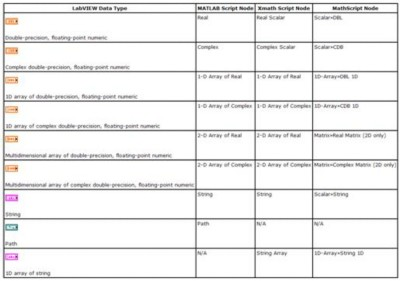Table 2. LabVIEW Data Types and Their Corresponding Data Types in the MATLAB, Xmath, and MathScript Script Nodes

The LabVIEW MathScript syntax in MathScript Nodes has the following format: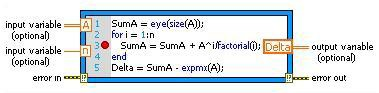Figure 7. LabVIEW MathScript Node Format

You can call functions from a MathScript Node. If you call a function from a MathScript Node that might change the MathScript search path list at run time or might introduce new variables at run time, a warning glyph (yellow sign), appears on the MathScript Node.

The following example uses a function for calculating the Fibonacci numbers as specified by the user. First, the G code version is presented; then, the LabVIEW MathScript version, which includes a warning glyph, is presented.

The Fibonacci numbers are computed according to the following algorithm: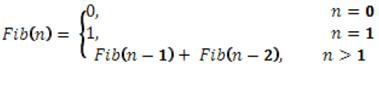Figure 8. Fibonacci Number Algorithm

The G programming language version is as follows: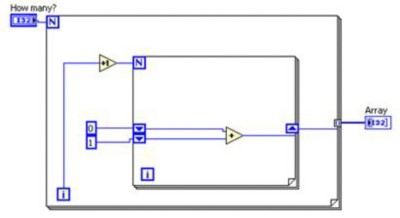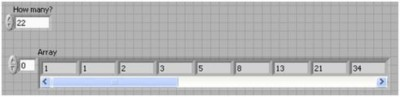Figure 9. Calculating Fibonacci Numbers in G Code

The equivalent LabVIEW MathScript code is as follows: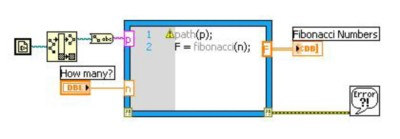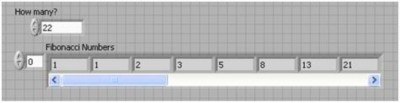Figure 10. Calculating Fibonacci Numbers in LabVIEW MathScript

The warning glyph in Figure 10 indicates a path change used for the.m file. Note that the LabVIEW Run-Time Engine and applications you deploy to real-time targets do not support MathScript Nodes with warning glyphs. If a VI includes a MathScript Node with a warning glyph, you must remove the warning glyph from the MathScript Node before you build a stand-alone application or shared library.

The above MathScript Node calls a function F, which is saved in a .m file with the following script: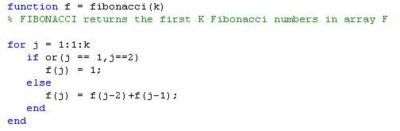You can create this file using the LabVIEW MathScript Window or an ASCII text editor such as the Microsoft Windows Notepad.

You also can combine the use of MathScript Nodes with certain LabVIEW modules and toolkits. For example, the LabVIEW Control Design and Simulation Module makes the state-space, transfer function, and zero-pole-gain models available for use in the MathScript Node (see Figure 11) and the Xmath script node. You must have the LabVIEW Control Design and Simulation Module installed to use these models.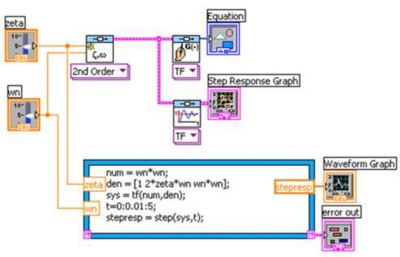Figure 11. LabVIEW MathScript Node Format

#### Scilab Script Node

Scilab is free software for numerical computation originally developed by researchers from the INRIA and the École nationale des ponts et chaussées (ENPC) in France  and now available from the Scilab Consortium (DIGITEO). It is a high-level programming language because most of its functionality is based on the ability to specify many computations with few lines of code. It does this primarily by abstracting primitive data types to functionally equivalent matrices.

As mentioned above, the Scilab script node uses a syntax similar to MATLAB, Octave, and Xmath. To use this node, you must install the Scilab scripting language on your computer. Also, you must install the Scilab LabVIEW Gateway software, available free of charge at ni.com, as described in this developer's zone document. After you install the software on your PC, the following menu is available within the LabVIEW development environment: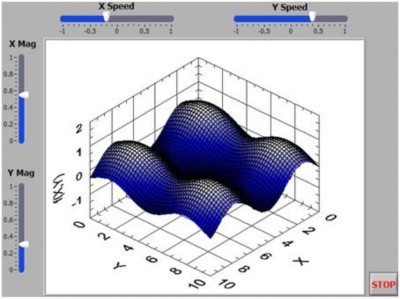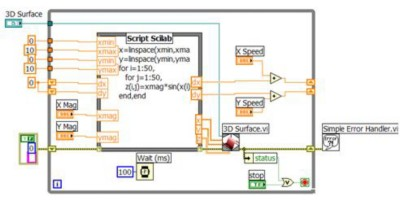Figure 12. Scilab Script Node Example

If you use LabVIEW applications and compiled code (EXEs) that incorporate the Scilab script node, you must make sure that the corresponding library of functions is installed on the machine where the application will run. LabVIEW depends on a DLL to connect to Scilab. As such, target machines that run stand-alone applications built with LabVIEW and that use the Scilab script node must include this DLL in the DLL support folder of the LabVIEW Run-Time Engine.

### Related Links

Learn more about LabVIEW MathScript

Download and install the LabVIEW / Scilab Gateway Software for the Scilab Script node

Scientific Computing with Graphical System Design

Introduction to Curve Fitting

https://forums.ni.com/t5/Example-Program-Drafts/Connecting-LabVIEW-with-Scilab/ta-p/3502031

Statistical Analysis

LabVIEW 2009 Help: Formula Node and Expression Node Functions

MATLAB® is a registered trademark of The MathWorks, Inc.

Back to top